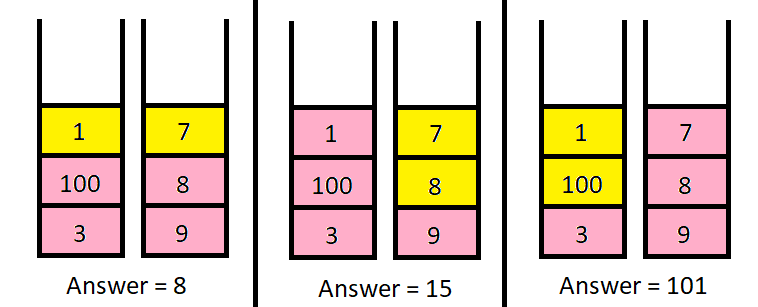# Maximum Value of K Coins From Piles Solution Leetcode

There are `n` piles of coins on a table. Each pile consists of a positive number of coins of assorted denominations.

In one move, you can choose any coin on top of any pile, remove it, and add it to your wallet.

Given a list `piles`, where `piles[i]` is a list of integers denoting the composition of the `ith` pile from top to bottom, and a positive integer `k`, return the maximum total value of coins you can have in your wallet if you choose exactly `k` coins optimally.

## Example 1: Maximum Value of K Coins From Piles Solution Leetcode```Input: piles = [[1,100,3],[7,8,9]], k = 2
Output: 101
Explanation:
The above diagram shows the different ways we can choose k coins.
The maximum total we can obtain is 101.
```

### Example 2: Maximum Value of K Coins From Piles Solution Leetcode

```Input: piles = [,,,,,,[1,1,1,1,1,1,700]], k = 7
Output: 706
Explanation:
The maximum total can be obtained if we choose all coins from the last pile.
```

## Constraints: Maximum Value of K Coins From Piles Solution Leetcode

• `n == piles.length`
• `1 <= n <= 1000`
• `1 <= piles[i][j] <= 105`
• `1 <= k <= sum(piles[i].length) <= 2000`